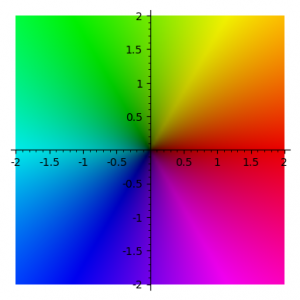# Quantum Fourier Transform

This demo shows the action of the quantum fourier transform (which is itself a version of the discrete fourier transform).

• TOP:  quantum state in register before QFT
• BOTTOM: quantum state in register after QFT

The complex coefficients of each classical state are represented as a coloured bar.  The colour represents the phase, using the usual domain colouring standard as in the picture below (red = positive real direction, greeny-yellow = positive imaginary direction).  The height represents the complex absolute value.You can alter the input function with your keyboard:

• N/n to increase/decrease the value of N
• A/a to increase/decrease the value of a
• B/b to increase/decrease the value of b
• C/c to increase/decrease the value of c
• Q/q to increase/decrease the number of qubits

The input function is $$f(x) = N\left \{ \frac{c + bx}{N} \right \} e^{2\pi i a x / N}$$